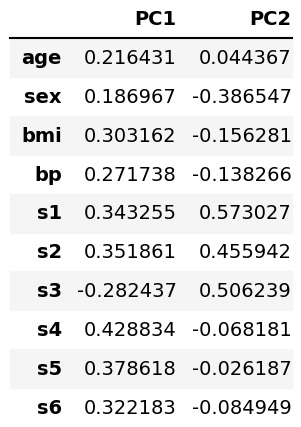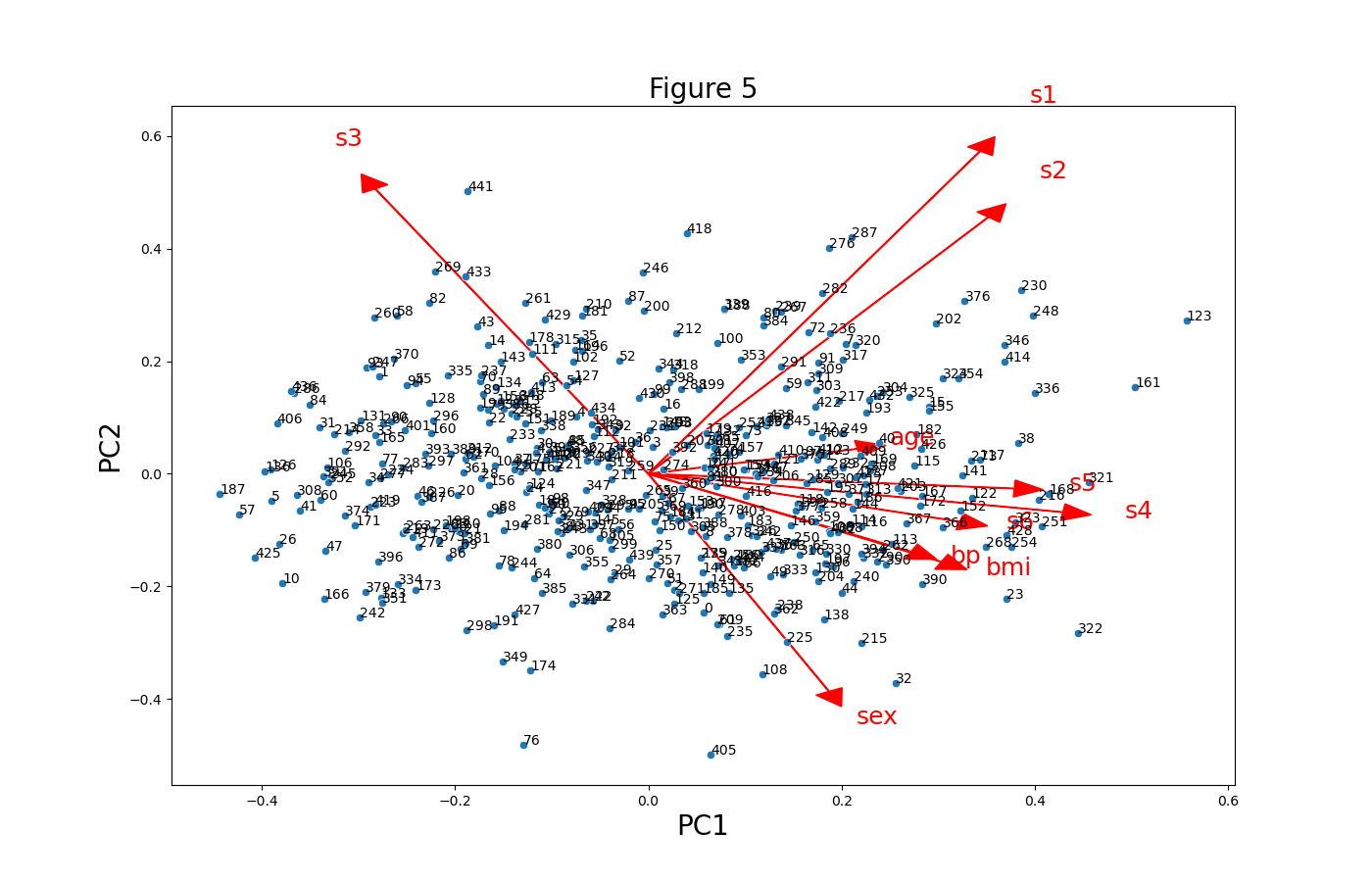# Draw Biplot of PCA in Python (3 Examples)

In this tutorial, you’ll learn how to create a biplot of a Principal Component Analysis (PCA) using the Python programming language.

This page was created in collaboration with Paula Villasante Soriano and Cansu Kebabci. Please have a look at Paula’s and Cansu’s author pages to get further information about their academic backgrounds and the other articles they have written for Statistics Globe.

Let’s get started!

## Example Data & Libraries

First, we will import some libraries to be used in the tutorial.

```import pandas as pd
import numpy as np
import matplotlib.pyplot as plt
import seaborn as sns
from sklearn.preprocessing import StandardScaler
from sklearn.decomposition import PCA```

Next, we will load the diabetes dataset containing ten features and a target column, which measures the disease progression in one year with respect to the baseline for 442 patients. Then, we will convert it into a DataFrame using the pd.DataFrame() function. After doing this, we will see what the first six rows of our data look like via the head() method.

```diabetes = load_diabetes()
df = pd.DataFrame(data=diabetes.data,
columns=diabetes.feature_names)As seen, the dataset was successfully loaded. Now, let’s perform our PCA!

## Scale Data and Perform PCA

Before performing the PCA, it’s important to scale our data to get better results. First, we will create a StandardScaler class object, then use it to fit our data matrix and transform the data into the new scale.

```scaler = StandardScaler()

scaler.fit(df)

Diabetes_scaled = scaler.transform(df)```

After this step, we will obtain the scaled data in a two-dimensional NumPy array with the exact dimensions as our original dataset.

Now, we can perform the PCA using the sklearn.decomposition.PCA algorithm. In this context, we will choose two principal components to perform our PCA, but see our tutorial Choose Optimal Number of Components for PCA if you want to learn how to select the ideal number of components in PCA.

`pca = PCA(n_components=2)`

After running the analysis, we can observe the principal component scores in a pandas DataFrame, as shown below. The scatter points in biplots show this data.

```PC_scores = pd.DataFrame(pca.fit_transform(Diabetes_scaled),
columns = ['PC 1', 'PC 2'])It is also possible to see the loadings in a DataFrame, as given below. The vectors in the biplots represent this data.

```loadings = pd.DataFrame(pca.components_.T, columns=['PC1', 'PC2'],
index=diabetes.feature_names)## Data of Biplot

In the previous section, we showed what kind of data of PCA is used in biplots. Now it is time to store them in variables before plotting. The arrays of the first and second principal components’ scores are saved under `PC1` and `PC2`, whereas the array of loadings is saved as `ldngs`.

```PC1 = pca.fit_transform(Diabetes_scaled)[:,0]
PC2 = pca.fit_transform(Diabetes_scaled)[:,1]
ldngs = pca.components_```

We should also define the scaling factors `scalePC1` and `scalePC2` to fit the data of `PC1`, `PC2` and `ldngs` on the same plot. Additionally, we can define the target group names to label the target groups in our biplots, see `features` below.

```scalePC1 = 1.0/(PC1.max() - PC1.min())
scalePC2 = 1.0/(PC2.max() - PC2.min())
features = diabetes.feature_names```

All are set and done, let’s start plotting!

## 1. Biplot Using Matplotlib

In this section we will show examples of how to create biplots using the Matplotlib library. Let’s check the first example!

### Example 1: Basic Biplot

In this example, we will create a basic biplot using a for loop to plot the loading vectors labeled per feature. Then, we will use the scatter() function to plot the scatter points.

```fig, ax = plt.subplots(figsize=(14, 9))

for i, feature in enumerate(features):
ax.arrow(0, 0, ldngs[0, i],
ldngs[1, i])
ax.text(ldngs[0, i] * 1.15,
ldngs[1, i] * 1.15,
feature, fontsize=18)

ax.scatter(PC1 * scalePC1,PC2 * scalePC2)

ax.set_xlabel('PC1', fontsize=20)
ax.set_ylabel('PC2', fontsize=20)
ax.set_title('Figure 1', fontsize=20)```

First, we loop through each feature of `features` that we have previously defined. Inside this loop, you can find the `ax.arrow()` function, which plots the vectors to represent the loadings per feature and the `ax.text()` function, which labels the feature names for each vector. Right after this, the `ax.scatter()` function plots the scatter points mapping the scaled PC1 and PC2 scores.Figure 1 is the visual we get by some default settings of Matplotlib. However, we can customize our biplot’s data points and loading vectors. Let’s see how to do it!

### Example 2: Customized Biplot with Labeled Points & Colored-Resized Vectors

The size and color of the loading vectors can be easily customized by adding the `head_width`, `head_length` and `color` arguments into the `ax.arrow()` function, as seen below.

```fig, ax = plt.subplots(figsize=(14, 9))

for i, feature in enumerate(features):
ax.arrow(0, 0, ldngs[0, i],
ldngs[1, i],
color="red")
ax.text(ldngs[0, i] * 1.15,
ldngs[1, i] * 1.15,
feature,color="red", fontsize=18)

ax.scatter(PC1 * scalePC1,
PC2 * scalePC2, s=5)

for i, label in enumerate(PC_scores.index):
ax.text(PC1[i] * scalePC1,
PC2[i] * scalePC2, str(label),
fontsize=10)

ax.set_xlabel('PC1', fontsize=20)
ax.set_ylabel('PC2', fontsize=20)
ax.set_title('Figure 2', fontsize=20)```

Similarly, we can add the `s` argument into the `ax.scatter()` function to resize the scatter points. However, we need to define a for loop to loop through `PC_scores.index` to label the scatter points iterating through 442 individuals.It would also be interesting to show the target groups of the dataset to see how they are segregated based on the new variables PC1 and PC2. Let’s see how to implement this in the next example!

### Example 3: Customized Biplot Colored By Target

We will color our scatter points by the target group in this example. Considering the high number of target groups, we will cluster them into equally sized three groups to get a better visualization. To do this, the `digitize()` function of NumPy will be called to divide the observations based on the given quantiles.

```target_groups = np.digitize(diabetes.target,
np.quantile(diabetes.target,
[1/3, 2/3]))```

As a result, we will get the `target_groups` array, which takes the values of 0, 1, or 2 indicating the group. Now we can plug it into the `ax.scatter()` function to color our biplot. Please also be aware that the color map `'viridis'` will be included in the function via the `cmap` argument. Many other color map options are available in Matplotlib if you are interested.

```fig, ax = plt.subplots(figsize=(14, 9))

for i, feature in enumerate(features):
ax.arrow(0, 0, ldngs[0, i],
ldngs[1, i],
ax.text(ldngs[0, i] * 1.15,
ldngs[1, i] * 1.15,
feature, fontsize = 18)

scatter = ax.scatter(PC1 * scalePC1,
PC2 * scalePC2,
c=target_groups,
cmap='viridis')

ax.set_xlabel('PC1', fontsize=20)
ax.set_ylabel('PC2', fontsize=20)
ax.set_title('Figure 3', fontsize=20)

ax.legend(*scatter.legend_elements(),
loc="lower left",
title="Groups")```

Note that we have also created a legend called `legend1`, which indicates the color-group matches, using ax.legend().We could also use the seaborn library to visualize our biplots. Let’s see how differently it operates!

## 2. Biplot of PCA Using seaborn

This section will show how to create biplots using the seaborn library. Let’s start with the first example!

### Example 1: Basic Biplot of PCA

Similar to the Matplotlib library, we will set a for loop to plot the vectors labeled per feature and then use a function to plot the scatter points.

```plt.figure(figsize=(14,9))

for i, feature in enumerate(features):
plt.arrow(0, 0, ldngs[0, i],
ldngs[1, i])
plt.text(ldngs[0, i] * 1.15,
ldngs[1, i] * 1.15,
feature, fontsize=18)

sns.scatterplot(x=PC1 * scalePC1,
y=PC2 * scalePC2)

plt.xlabel('PC1', fontsize=20)
plt.ylabel('PC2', fontsize=20)
plt.title('Figure 4', fontsize=20)```

First, we loop through each feature of `features` and run the `plt.arrow()` function, which plots the vectors to represent the loadings per feature and the `plt.text()` function, which labels each vector by the feature name. Right after this, the `sns.scatterplot()` function plots the scatter points mapping the scaled PC1 and PC2 scores.Now, let’s see how to label the scatter points and change the color and size of the loading vectors in the next example!

### Example 2: Customized Biplot with Labeled Points & Colored-Resized Vectors

The code structure in Example 2 of the first section could be adapted to customize the scatter and vectors in seaborn. Briefly, the `sns.scatterplot()` function should be called, and the location of the functions should be defined as `plt` instead. See the code script below.

```plt.figure(figsize=(14,9))

for i, feature in enumerate(features):
plt.arrow(0, 0, ldngs[0, i],
ldngs[1, i],
color="red",
plt.text(ldngs[0, i] * 1.15,
ldngs[1, i] * 1.15,
feature,
color="red",
fontsize=18)

sns.scatterplot(x=PC1 * scalePC1,
y=PC2 * scalePC2)

plt.xlabel('PC1', fontsize=20)
plt.ylabel('PC2', fontsize=20)
plt.title('Figure 5', fontsize=20)

for i, label in enumerate(PC_scores.index):
plt.text(PC1[i] * scalePC1,
PC2[i] * scalePC2, str(label),
fontsize=10)```Next, we will replicate the colored biplot in the first section using the seaborn library. Let’s see how it is done in the next example!

### Example 3: Customized Biplot Colored by Target

Also, in this case, we can adapt the code of Example 3 in the first section to color the biplot by the target using the seaborn library. You can see how the `hue` argument is set to `target_groups` below.

```plt.figure(figsize=(14,9))

for i, feature in enumerate(features):
plt.arrow(0, 0, ldngs[0, i],
ldngs[1, i],
plt.text(ldngs[0, i] * 1.15,
ldngs[1, i] * 1.15,
feature, fontsize=18)

sns.scatterplot(x=PC1 * scalePC1,
y=PC2 * scalePC2,
hue=target_groups,
palette="viridis")

plt.xlabel('PC1', fontsize=20)
plt.ylabel('PC2', fontsize=20)
plt.title('Figure 6', fontsize=20)```

Again, we have used the “viridis” palette, but feel free to choose the one that looks more appropriate in your case.We have shown different customizations of biplots in Python using the Matplotlib and seaborn libraries. If you are interested in different ways and motives to visualize your PCA results, we recommend you to check our tutorial Visualization of PCA in Python. See you next time!

## Video, Further Resources & Summary

Do you need more explanations on the steps and application of a Principal Component Analysis in Python? Then you should look at the following YouTube video of the Statistics Globe YouTube channel.

Please accept YouTube cookies to play this video. By accepting you will be accessing content from YouTube, a service provided by an external third party.If you accept this notice, your choice will be saved and the page will refresh.

There are other tutorials on Statistics Globe you could have a look at:

This post has shown how to create biplots for PCA in Python. If you have further questions, don’t hesitate to comment.

Subscribe to the Statistics Globe Newsletter